PMG = Parallel algebraic MultiGrid

Overview PMG is an algebraic multilevel code written in several languages (FORTRAN, C, C++, and CC++). The original FORTRAN/C/C++ versions were written for sequential computers, whereas the more recent CC++ port is a distributed memory parallel implementation.

All of the versions of PMG are designed to numerically approximate the solutions of linear and nonlinear elliptic partial differential equations in three-dimensional (logically) brick-like domains in an extremely efficient and robust way. To accomplish this task, PMG employs a non-uniform Cartesian mesh of the user's choice, box-methods or finite element methods for discretization, algebraic (or geometric) construction of lower-dimensional subspace problems, damped-inexact Global Newton methods for nonlinearities, and a jacobian multilevel iteration based on the algebraic (or geometric) subspace hierarchy.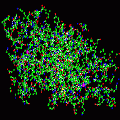The code is applicable to three-dimensional nonlinear Poisson-like scalar equations, allowing for nonlinearities that depend on the scalar unknown (but not on derivatives); nonlinearities of this type occur in the Poisson-Boltzmann equation as well as in other applications, such as the drift-difussion equations in semiconductor modeling. The class of problems for which the code is applicable can be extended with suitable modifications to the code (the methods need not be modified).

For strongly elliptic equations with self-adjoint differential operators and smooth coefficients, the solution method is provably optimal (meaning O(N), where N mesh points are used on the finest user-specified mesh). For strongly elliptic self-adjoint operators with simple coefficient discontinuities, the solution method is provably O(N log N). For strongly elliptic self-adjoint operators with arbitrary discontinuities, the solution method is provably convergent, and emperically appears to retain the O(N log N) behavior.

At the heart of the code is the algebraic construction of the lower-dimensional subspace problems, which is performed by a Maple-generated code. This code was generated by Maple using a symbolic stencil calculus.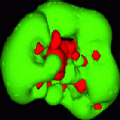Usage

To use the code as provided, the user defines the problem coefficients, the mesh points lying on the finest non-uniform Cartesian mesh along with the basic domain geometry, as well as the boundary conditions and types, in a very simple and well-defined way. All necessary workspace is passed in by the user; the code will indicate exactly how much workspace is required for a particular problem configuration. The nonlinearity must be defined in a subroutine supplied by the user which is to be called by the code, and a second subroutine must also be supplied which provides the derivative of the nonlinear term for use in forming the Jacobian required by the Newton solver. Using the solver involves a single subroutine call with a simple and understandable argument list.

Discretization

The code is restricted to ``logically'' non-uniform Cartesian three-dimensional meshes due to the multilevel solver employed, and the box-method discretization routine we provide requires a ``physically'' non-uniform Cartesian mesh as well. This discretization routine allows arbitrary Dirichlet or Neumann boundary conditions at any boundary point on a three-dimensional rectangular box, allowing for specification of arbitrary contact placements and geometries, as may be necessary in semiconductor modeling. Although we provide a box-method discretization with the code, the multilevel method can form the coarse level equations algebraically, the only requirement on the fine level discretization being that the equations be representable as stencils, and that the nonlinear term be diagonal. This is always true for either box or finite element (with mass lumping) discretization of the above class of equations on a logically non-uniform Cartesian mesh.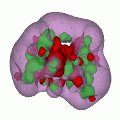Algebraic and geometric construction of coarse problems

The linear multilevel method at the core of the code is designed specifically for discontinuous coefficients as occur in material interface problems. Techniques employed include coefficient averaging methods or algebraric (Galerkin) methods, combined with operator-induced prolongation procedures which attempt to enforce flux conservation at box boundaries when a grid function is interpolated from a coarse to a fine mesh. The averaging methods are usually sufficient for many problems (such as the Poisson-Boltzmann equation), but particularly complex discontinuities (i.e., complex molecular surfaces) seem to require the Galerkin approach for robustness; this is computationally more costly and requires more memory than the averaging approach (seven-diagonal matrices produced by the box-method on the fine mesh expand to twenty-seven-diagonal matrices on coarse meshes), but it always converges rapidly. Galerkin coefficient expressions are extremely complex in three dimensions, and were obtained with the help of the MAPLE symbolic manipulation package.

Multilevel linear solver

The user is given many choices in configuring the multilevel method, including choice of smoother, number of levels employed, iteration strategy, choice of prolongation operator, coarse problem formulation, as well as iterative (CG) or direct (banded LINPACK) solve on the coarsest mesh. A conjugate gradient method is also employed to accelerate the multilevel methods, and while this approach is mathematically guaranteed to improve the convergence rate of the multilevel method with a corresponding increase in computational labor, it is also the case that in practice the CPU time to solve the problem is usually reduced using the acceleration (i.e., the acceleration more than pays for itself).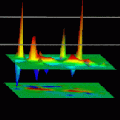Nonlinear solver

Nonlinear problems are handled with a robust (essentially globally convergent) inexact-Newton solver coupled with the fast linear multilevel method. Configuration options include a choice of several stopping criteria, degree of inexactness in the approximate Newton solves, and damping strategy, in addition to all possible configuration options for the multilevel Jacobian system solver. A nonlinear operator-based prolongation procedure has been developed for the nonlinear case as well. Also included in the code are several implementations of more traditional nonlinear methods, including nonlinear SOR (SOR-outer iteration with one-dimensional Newton-solves), nonlinear CG (the Flether-Reeves nonlinear extension to the linear Hestenes-Steifel algorithm), and a ``true'' nonlinear multilevel method (the ``FAS'' or ``nonlinear multigrid'' algorithm).

Poisson-Boltzmann equation-specific code

An interface routine is provided for using the code specifically as a nonlinear Poisson-Boltzmann equation solver. To handle the severe numerical problems occurring with nonlinearities of exponential-type present in the Poisson-Boltzmann equation, we developed argument-capping functions which avoid nonvectorizable statements. Calls to the standard intrinsic functions are replaced by these modified functions, and overflows are successfully avoided during early transient iterations without loosing the execution efficiency of the intrinsic functions.

The C/C++/CC++ version and parallel computing

The C/C++/CC++ version of PMG runs sequentially or in parallel on a wide range of hardware. Parallelism is obtained on workstation clusters as well as supercomputers such as the SP2 through the CC++ (Compositional C++) extensions.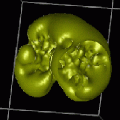The (sequential) FORTRAN 77 version

There is also a sequential FORTRAN 77 version of PMG (which we refer to as MG) with much of the core numerical capabilities as the C/C++/CC++ version. The F77 version installs itself in single or double precision on the specified machine, inserting the appropriate compiler directives automatically, and runs without modification on most current systems, including the Cray C90, Cray Y-MP, Convex C3, Convex C240, Convex C220, IBM RS/6000, Sun 4, Sun 3, and most other systems with a standard FORTRAN 77 compiler.

The X Interface in C

An X-interface has been written (in C) for running the numerical code. The interface is completely disconnected from the numerical code in the sense that it provides only input files which are read by the numerical code, executes the numerical code, and then reads and processes the output files produced by the numerical code. The X-interface runs as is on any machine that has a standard C compiler and the standard X11 distribution Release 4 or newer. The interface uses only the Athena Widget set (along with Xlib and the Xt intrinsics), so that the interface is also quite portable.

Obtaining PMG

The C++ version of PMG was developed by Michael Holst at Caltech, based on his initial FORTRAN version developed at the University of Illinois. The CC++ extension of PMG was developed by John Garnett and Carl Kesselman at Caltech. PMG is copylefted and distributed under the GNU General Public License (GPL). (A copy of the GPL should be included with your copy of PMG.)